FreeBuf+小程序GSM Sniffing嗅探设备组装之短信嗅探
2019-02-21 08:00:26

## 0x1 设备清单

1. 摩托罗拉 C118手机 （6个改装过）

2. 树莓派板

3. USB拓展板 （6口的）

4. USB 转串口 FT232rl (6个)

5. 电源变压器一个

6. 自定义电源中心控制器一个

7. 天线 6根

8. 承载用盒子一枚

9. N个开关

10. N个电阻和针脚

11. 小号信号降频设备 （测试用）

12. 6类网线或者其他类型的线。（串联设备用）

13. 锡焊机器 (焊接设备用)

14. 无线wifi路由器 (调试用)

## 0x2 设备安装

### 1. 改装C118手机

C118手机是不可缺少的一部分，为什么要用6个设备呢？因为一台c118手机只能够监听也就是嗅探一个频点，所以按道理来说手机越多越好。来一个改装过全图，下图中是我做好的C118手机，仅供参考，实际情况看你自己需求。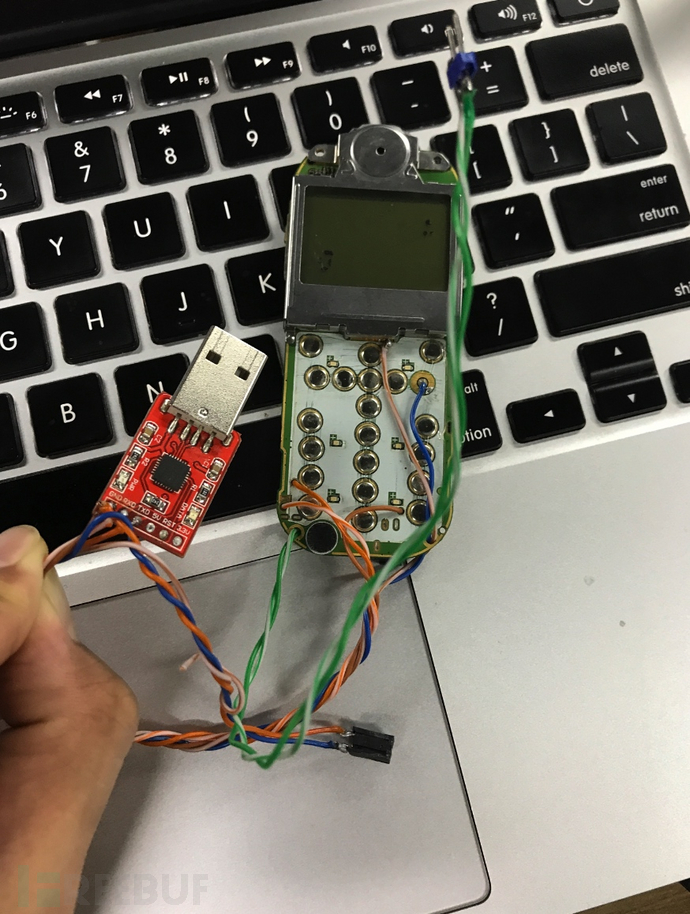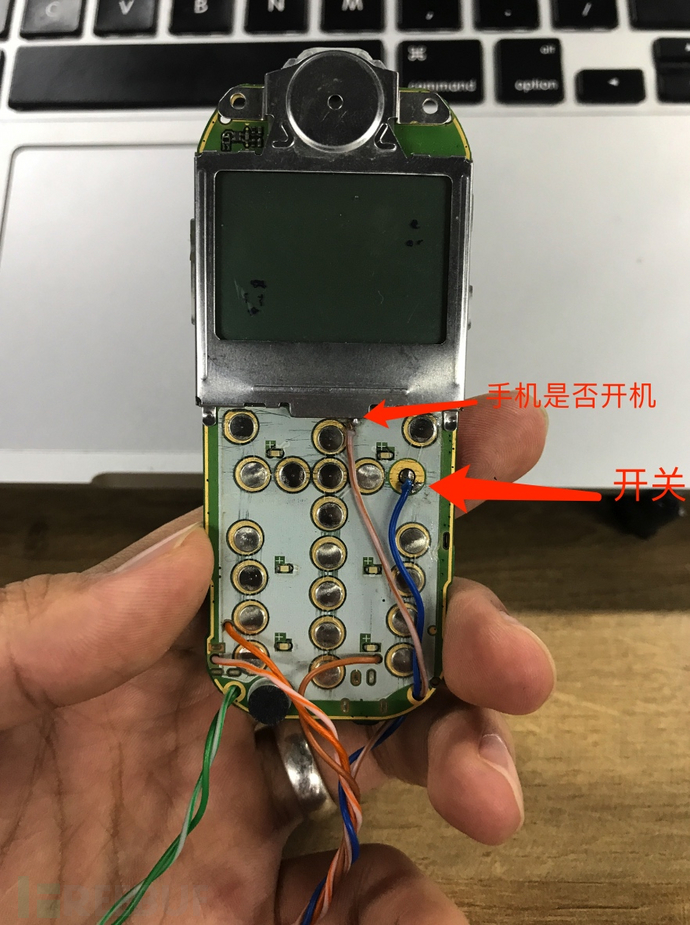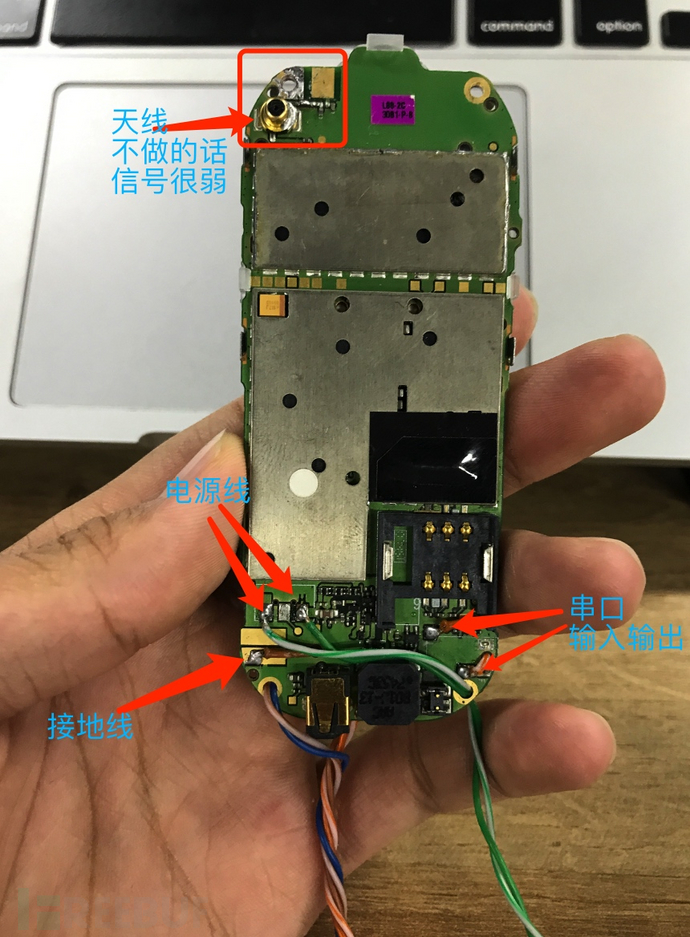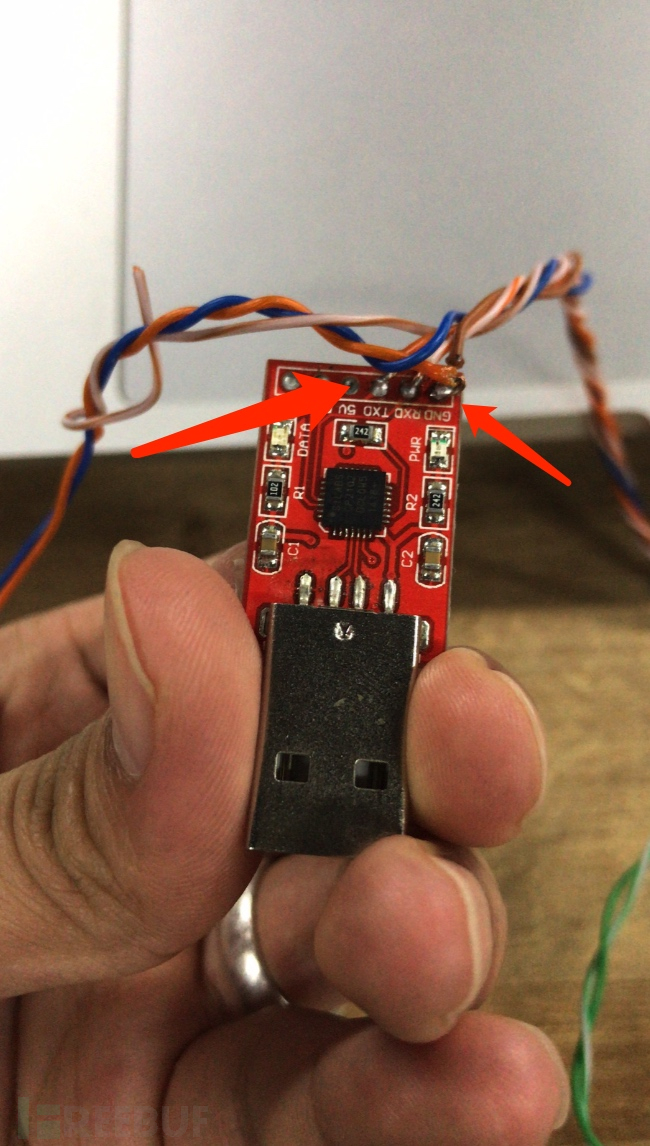usb串口转接线 一定别接错了 否则无法开机无法刷机。。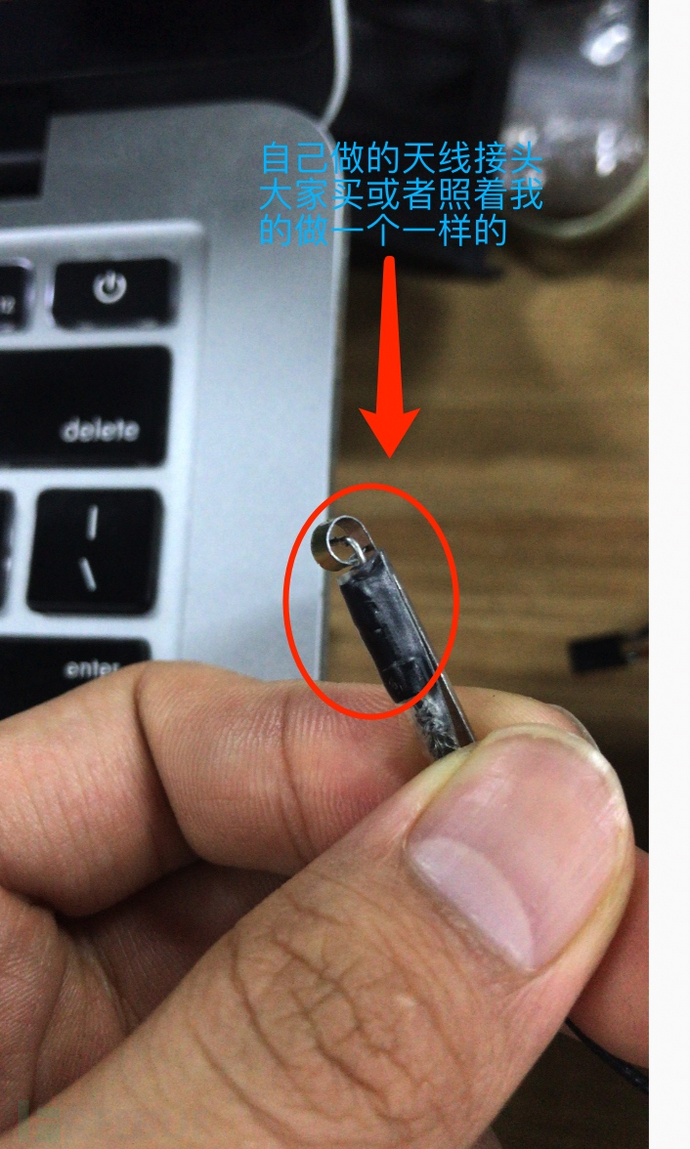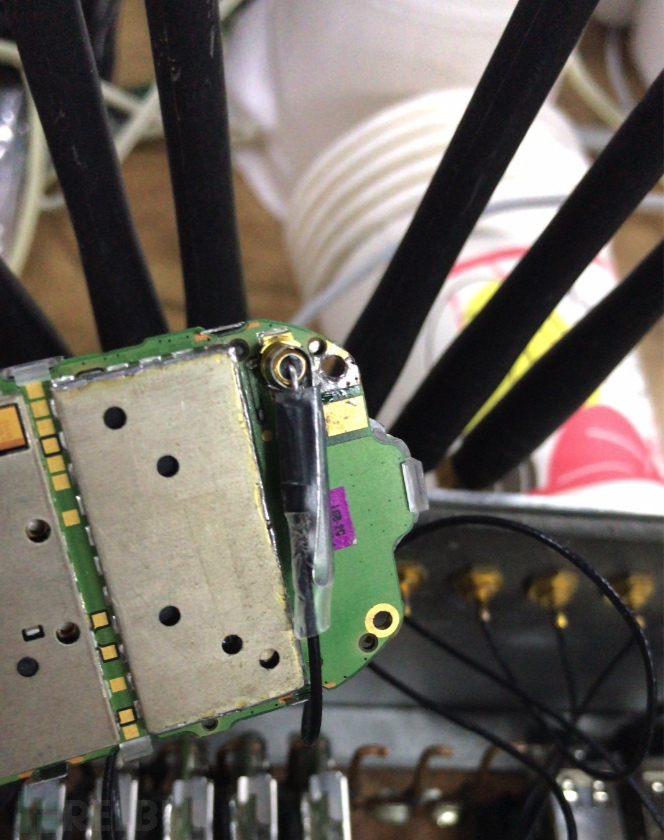### 2. 组装

2.1 供电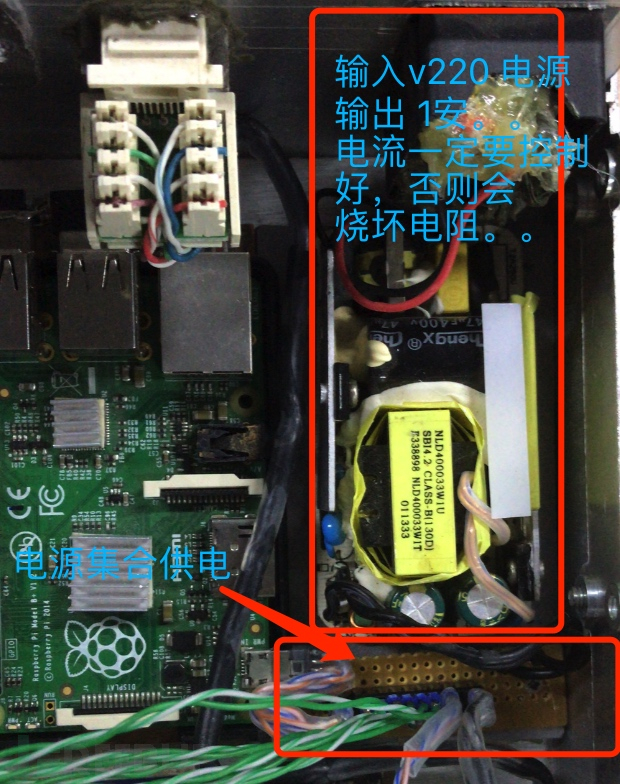2.2 树莓派和USB集线器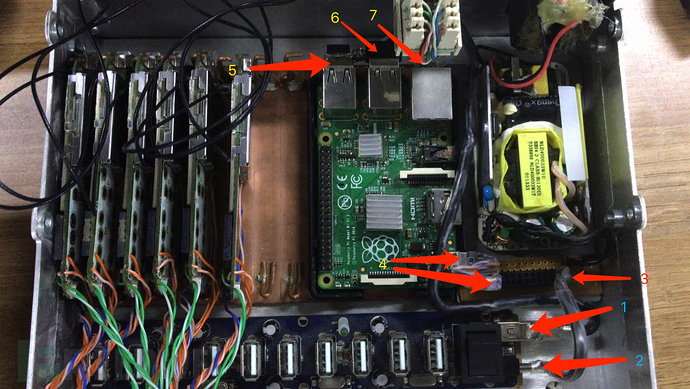1. USB集线器数据线接到树莓派USB口

2. USB集线器电源接到供电开发板上

3. USB集线器电源接口

4. 树莓派供电口

5. 树莓派接集线器USB接口

6. 树莓派USB接的无线网卡

7. 树莓派网口接的网线转接头

2.3 电源开关控制器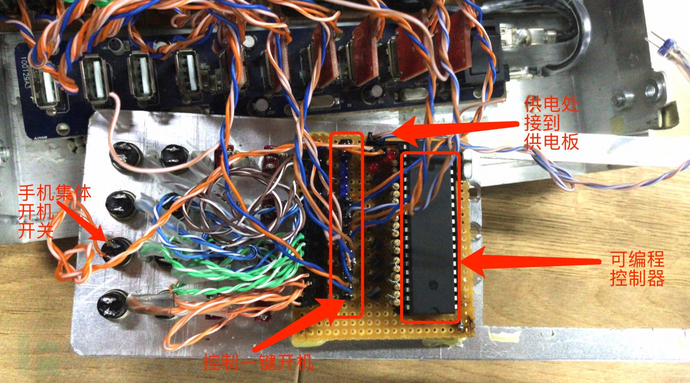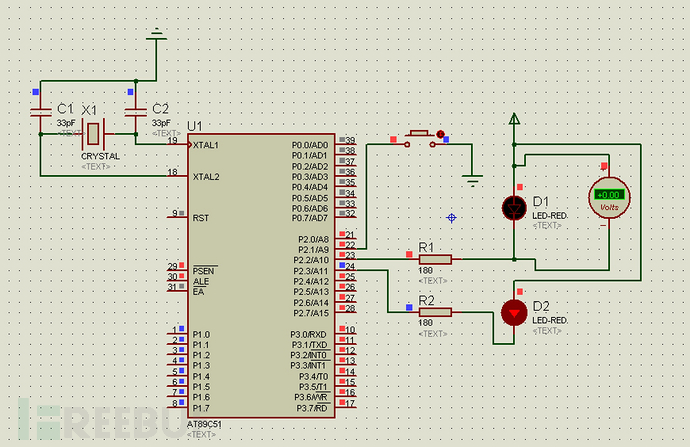C52单片机代码

``````#include <reg52.h>

void delay(unsigned int);
sbit LED0 = P0^0;
sbit LED1 = P0^1;
sbit LED2 = P0^2;
sbit LED3 = P0^3;
sbit LED4 = P0^4;
sbit LED5 = P0^5;
sbit LED6 = P0^6;
sbit LED7 = P0^7;
sbit LED_0 = P1^0;
sbit LED_1 = P1^1;
sbit LED_2 = P1^2;
sbit LED_3 = P1^3;
sbit LED_4 = P1^4;
sbit LED_5 = P1^5;
sbit LED_6 = P1^6;
sbit LED_7 = P1^7;
sbit STAU_A = P2^3;
sbit STAU_B = P2^2;
sbit KEY = P2^1;

void delay(unsigned int i){
unsigned int j,k;
while(i--){
for(j = 0; j < 255; j++)
for(k = 0; k < 240; k++);
}
}

void main(){
unsigned char i;
while(1){
i=KEY;
STAU_A=0;
if(i==0){
i=KEY;
if(i==0)
STAU_B = 0;
LED0 = 0;
LED1 = 0;
LED2 = 0;
LED3 = 0;
LED4 = 0;
LED5 = 0;
LED6 = 0;
LED7 = 0;
LED_0 = 0;
LED_1 = 0;
LED_2 = 0;
LED_3 = 0;
LED_4 = 0;
LED_5 = 0;
LED_6 = 0;
LED_7 = 0;
delay(10);
STAU_B=1;
LED0 = 1;
LED1 = 1;
LED2 = 1;
LED3 = 1;
LED4 = 1;
LED5 = 1;
LED6 = 1;
LED7 = 1;
}else{
i=KEY;
}
}
}``````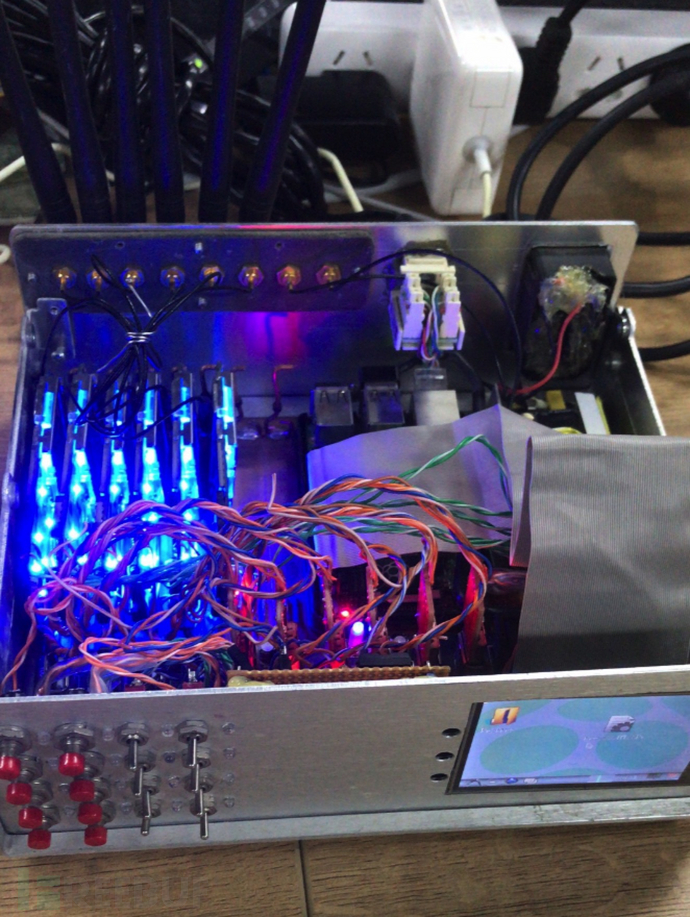## 0x3 设备调试

### 1. 树莓派调试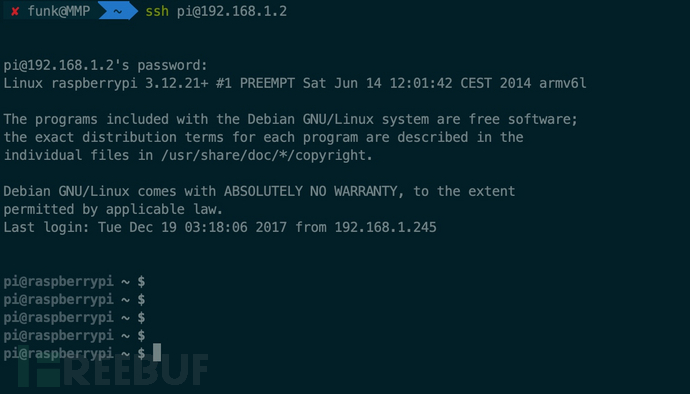### 2. 编译

``sudo apt-get install libtool shtool autoconf git-core pkg-config make gcc``

gnuarm:

``32 bit wget http://www.gnuarm.com/bu-2.16.1_gcc-4.0.2-c-c++_nl-1.14.0_gi-6.4_x86-64.tar.bz2 tar xf bu-2.16.1_gcc-4.0.2-c-c++_nl-1.14.0_gi-6.4_x86-64.tar.bz2 mv gnuarm-* ~/gnuarm export PATH=~/gnuarm/bin:\$PATH``

libosmocore:

``git clone git://git.osmocom.org/libosmocore.git cd libosmocore/ autoreconf -i./configuremakesudo make installcd ..ldconfig``

https://github.com/K1two2/OsmocomBB_Raspberry-Pi_SMS_WEB_CODE

### 3. 确定当前手机接入基站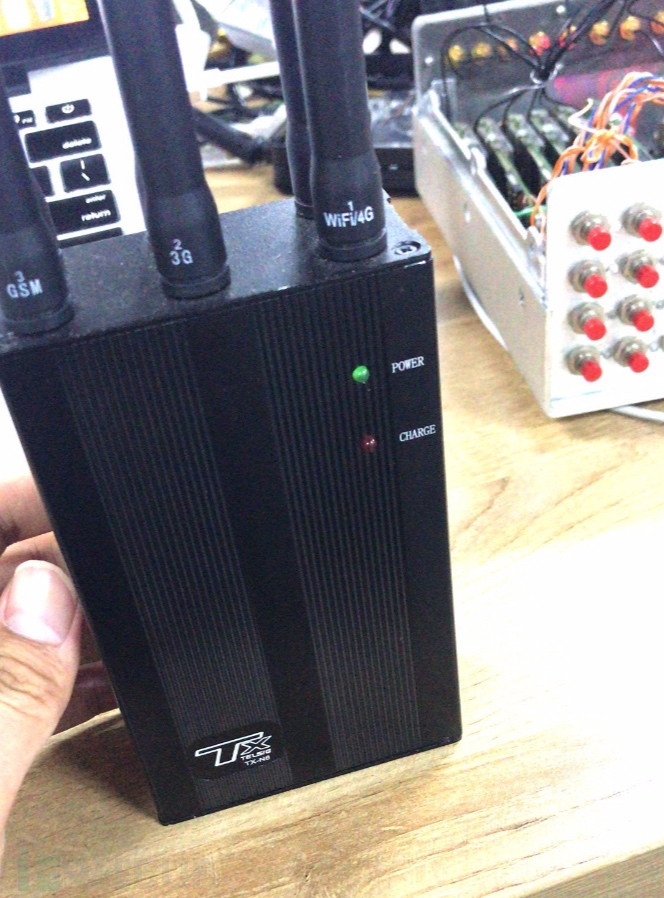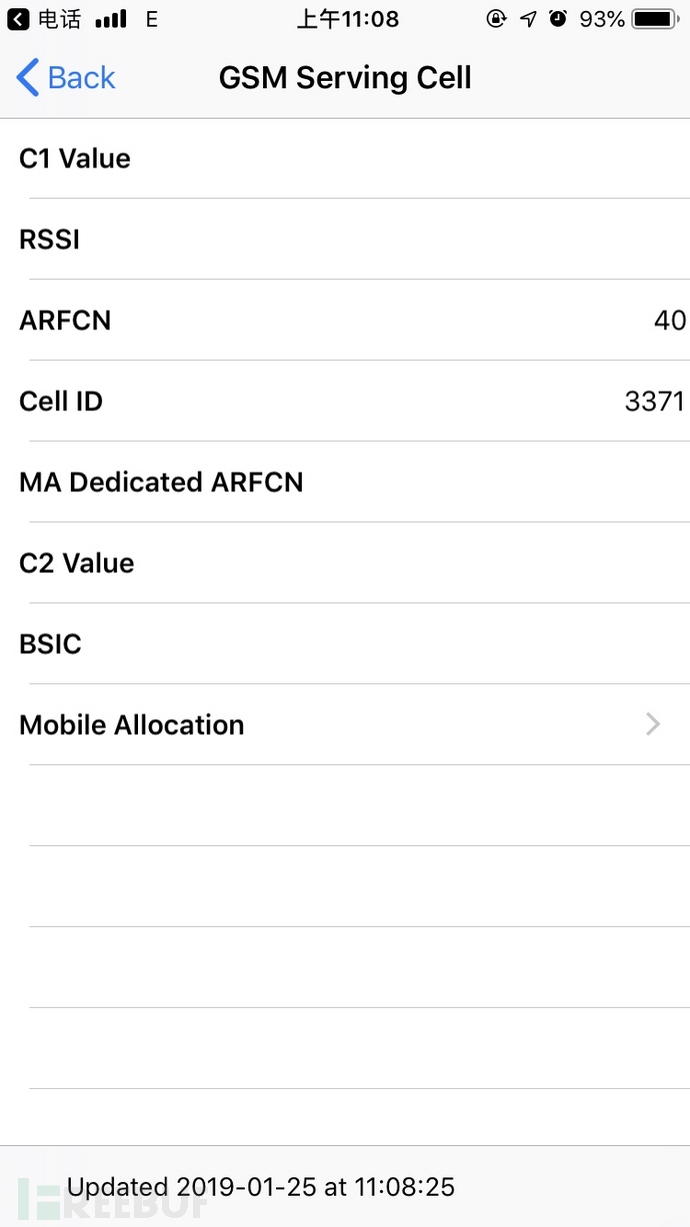``````Samsung (Android) : *#*#197328640#*#* or *#0011#
iPhone (all) : *3001#12345#* 拨号
HTC (Android) : *#*#7262626#*#*
``````

``````MCC 移动国家码

MNC Mobile Network Code，移动网络码，共2位，中国联通GSM系统使用01，中国移动GSM系统使用02

ARFCN 绝对无线频道编号（Absolute Radio Frequency Channel Number – ARFCN ），是在GSM无线系统中，用来鉴别特殊射频通道的编号方案。

### 4. 测试设备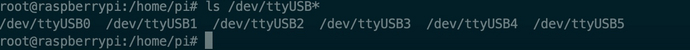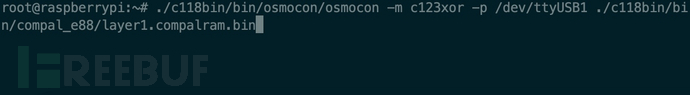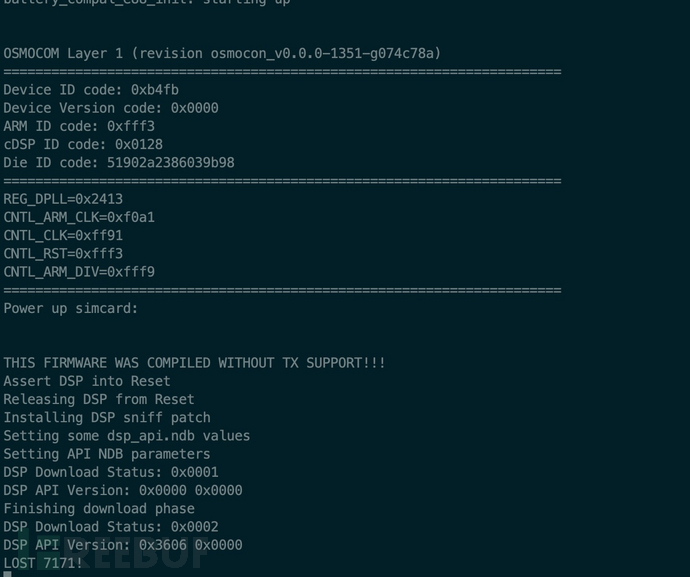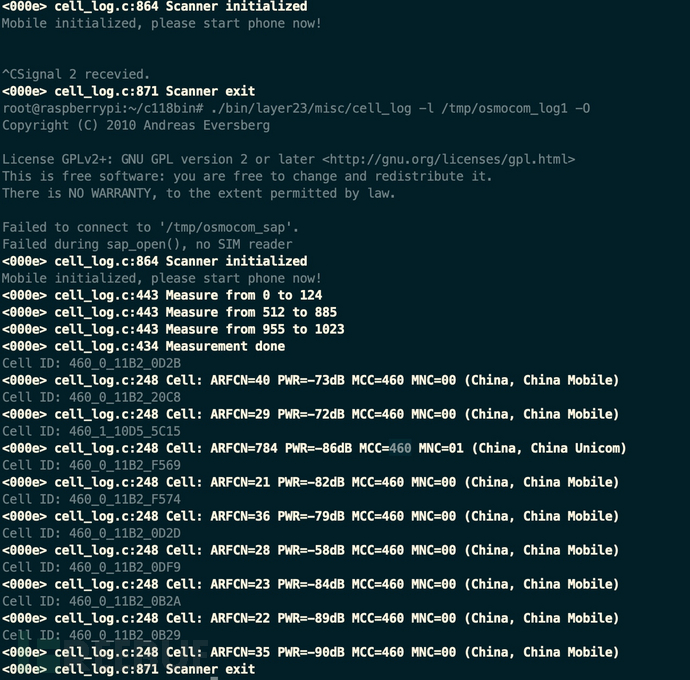``sudo wireshark -k -i lo -f 'port 4729'``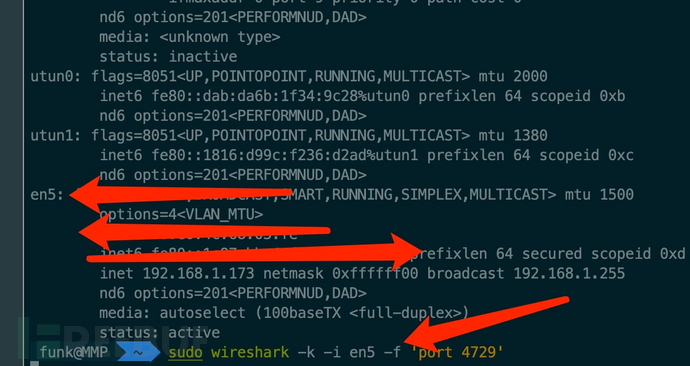/root/c118bin/bin/layer23/misc/ccch_scan -i 你的外网地址 -a 频段：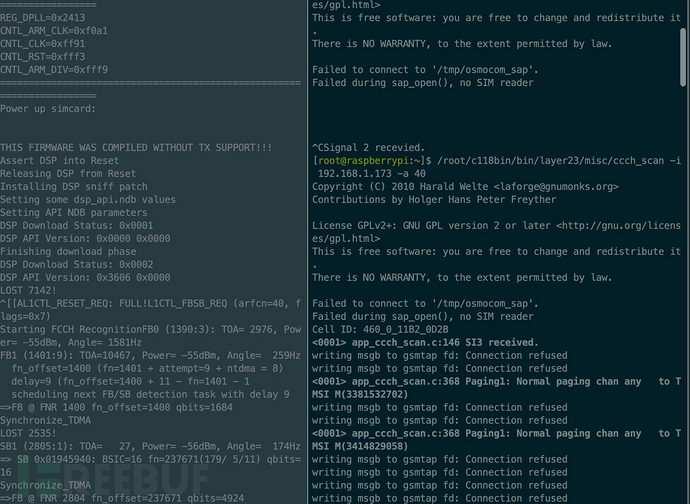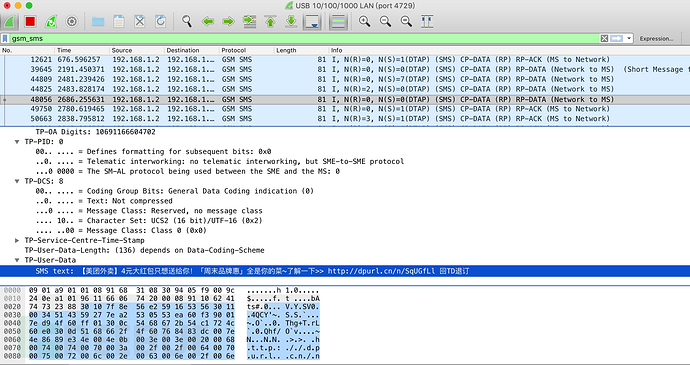### 5. 开车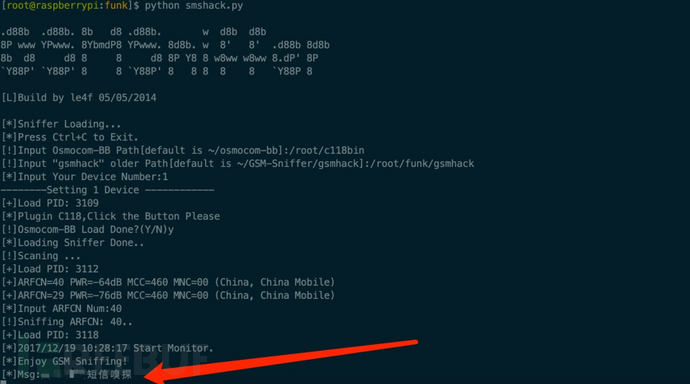## 0x4 结尾

GSM嗅探不算什么最新技术，但是是一个无线安全的必经之路，该文章单为记录操作过程，方便以后观看，设备也是花了不少时间才做完。

## 0x5 参考文献

https://github.com/K1two2/OsmocomBB_Raspberry-Pi_SMS_WEB_CODE

https://github.com/lxf94/GSM-Sniffer

https://www.freebuf.com/geek/160171.html

http://www.ver007.org

*本文作者：安百科技，转载请注明来自FreeBuf.COM

# 短信嗅探 # GSM Sniffing

+ 收入我的专栏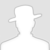• 0 文章数
• 0 评论数
• 0 关注者